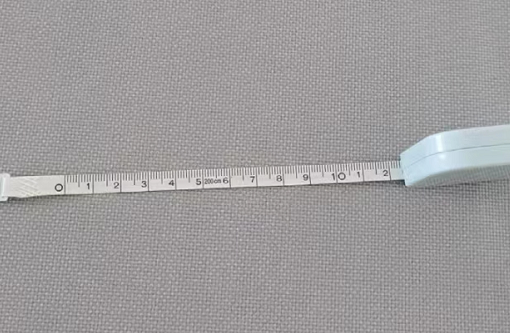# 腰围尺码表怎么算？标准三围怎么算？29码=2.2尺腰=73.3cm

30码=2.3尺腰=76.7cm

31码=2.4尺腰=80cm

32码=2.5尺腰=83.3cm

33码=2.6尺腰=86.7cm

34码=2.7尺腰=90cm

36码=2.8尺腰=93.3cm

38码=2.9尺腰=96.7cm

40码=3.0尺腰=100cm